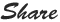# PHP Assignment Operators

Assignment Operators in PHP

Assignment operators mainly used to assign one value in other variable or assign one variable value to other variable.

Or this can be used to make a calculation from one value with other value.  Find below table with example . Fist column is the name of the operator second column is the sign of the operator and third is the description with example.

 Operator Sign Description Addition + To make a sum of two or more than two values Example : Output : 30 Subtraction - To find a difference between two or more than two values.  Example : Output : 10 Multiplication * To perform a Multiplication between two or more than two values Example : Output : 200 Division / To perform division between two values where as one value is Quotient and other value is divider of that Quotient values Example : Output : 2 Modulus % To find the reminder after division operation, so modules operation performs the divide operation internally and it shows the reminder Example : Output : 2 i.e.   10/4   Then   4*1=4,    4*2=8 So will keep  8 as its less than 10 and closer to 10 , then the reminder is 2    ( 10-8 = 2)